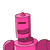# evaluate alpha^-1 + beta^-1 if alpha and beta are the zeros of polynomial f(x)=9x^2-3x-2​

evaluate alpha^-1 + beta^-1 if alpha and beta are the zeros of polynomial f(x)=9x^2-3x-2​

### 1 thought on “evaluate alpha^-1 + beta^-1 if alpha and beta are the zeros of polynomial f(x)=9x^2-3x-2​”

1.Step-by-step explanation:

f(x) = 9x²-3x-2

acc to ques , alpha and beta is the zeros of polynomial f(x)

so, alpha + beta = -(-3)/9 = 1/3 and apla × beta = (-2)/9

we have to find 1/(apha) + 1/(beta)

= [(apha) + (beta)]/(apha)×(beta)

= 1/3÷(-2)/9